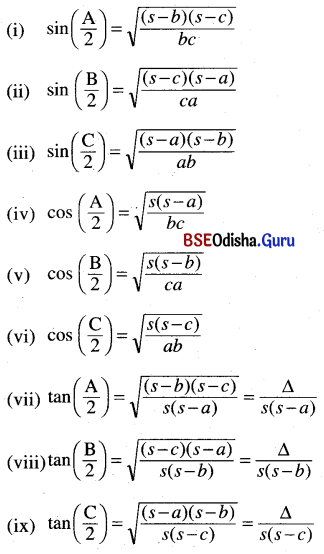# CHSE Odisha Class 11 Math Notes Chapter 4 Trigonometric Functions

Odisha State Board CHSE Odisha Class 11 Math Notes Chapter 4 Trigonometric Functions will enable students to study smartly.

## CHSE Odisha 11th Class Math Notes Chapter 4 Trigonometric Functions

Angle:
If A, B, and C are three non-collinear points, then ∠ABC = $$\overrightarrow{\mathrm{BA}} \cup \overrightarrow{\mathrm{BC}}$$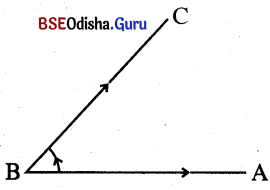$$\overrightarrow{\mathrm{BA}}$$ is the initial side, $$\overrightarrow{\mathrm{BC}}$$ is called the terminal side and B is called the vertex of the angle.

Positive and negative angles:
If the direction of rotation is anti-clockwise then the angle is positive and if the direction of rotation is clockwise then the angle is negative.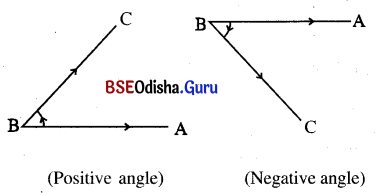Measure of an angle:
(a) Sexagesimal system or English System (Degree measure):
1 degree = 1° = $$\left(\frac{1}{360}\right) \text { th }$$ of revolution from initial side to terminal side.

• One revolution = 360°
• 1° = 60′ (sixty minute)
• 1′ = 60” (sixty seconds)

One radian = 1c = The angle at the centre of the circle by an arc where the arc length equals to

Note:
(i) θ = $$\frac{l}{r}=\frac{\text { arc }}{\text { radius }}$$ where θ is an radian.
(ii) θ in radian is created as a real number.

(c) Relation between Degree and radian measure:

• We can convert radian to degree or degree to radian by using the identity.
$$\frac{\mathrm{D}}{180}=\frac{\mathrm{R}}{\pi}$$ where D is the degree measure and R is the radian measure of an angle.Trigonometry Functions: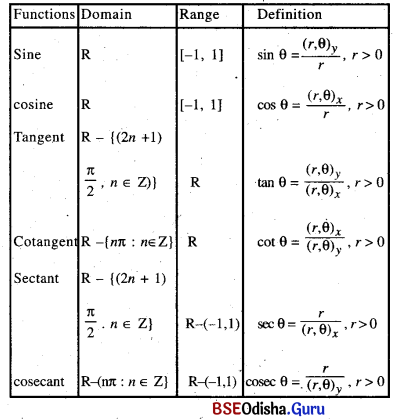(i) Sign of trigonometry functions: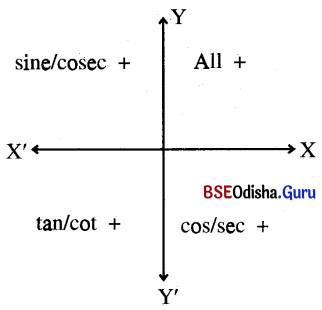(ii) $$\begin{array}{cccc} \text { Add } & \text { Sugar } & \text { To } & \text { Coffee } \\ \downarrow & \downarrow & \downarrow & \downarrow \\ \text { all }+ & \sin + & \tan + & \cos + \end{array}$$

(iii) Periodicity of trigonometry functions:

 Trigonometric function Period sin x 2π cos x 2π tan x π cot x π sec x 2π cosec x 2π sin2 x or cos2 x π |sin x| or |cos x| π

Trigonometric functions of some standard angles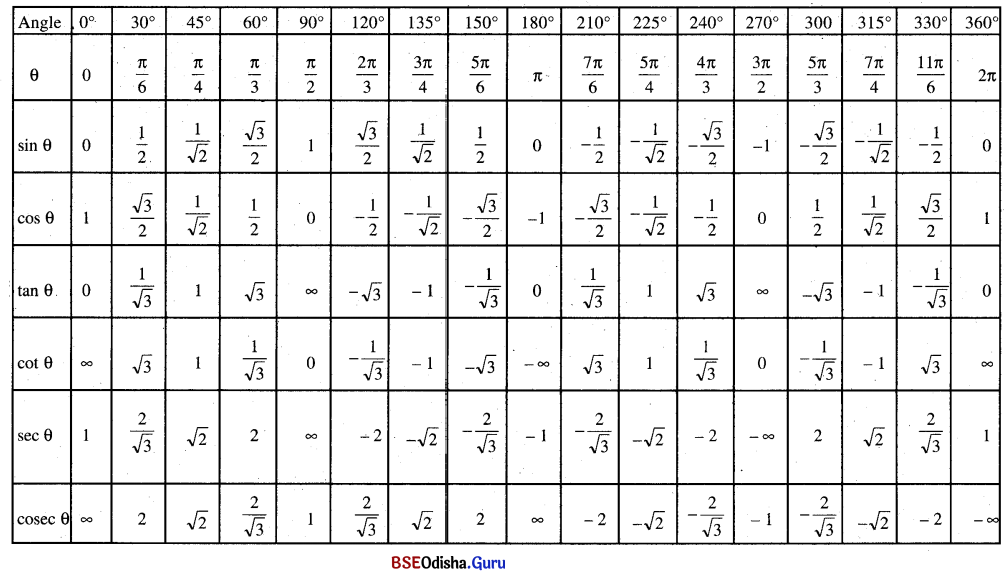Fundamental trigonometric identities:
(a) sin θ = $$\frac{1}{{cosec} \theta}$$
(b) cos θ = $$\frac{1}{{sec} \theta}$$
(c) tan θ = $$\frac{1}{{cot} \theta}$$
(d) sin2 θ + cos2 θ = 1
(e) sec2 θ – tan2 θ = 1
(f) cosec2 θ – cot2 θ = 1
(g) sin (-θ) = -sin (θ)
(h) cos (-θ) = -cos (θ)

Trigonometric functions of allied angles:
(a) sin $$\left((2 n+1) \frac{\pi}{2} \pm \theta\right)$$ = (±) cos θ choose + or – in (±) by using ASTC rule

(b) cos $$\left((2 n+1) \frac{\pi}{2} \pm \theta\right)$$ = (±) sin θ choose + or – in (±) by using ASIC rule
Similar technique can be used for other trigonometric functions.

(c) sin (nπ ± θ) = (±) sin θ
cos (nπ ± θ) = (±) cos θ
tan (nπ ± θ) = (±) tan θ
choose + or – in (±) by using ASTC rule.

Sum And Difference Formulae:
(a) sin(A + B) = sin A . cos b + cos A . sin B

(b) sin(A – B) = sin A . cos B – cos A . sin B

(c) cos(A + B) = sin A . cos B – cos A . sin B

(d) cos(A – B) = sin A . cos B + cos A . sin B

(e) tan(A + B) = $$\frac{\tan A+\tan B}{1-\tan A \cdot \tan B}$$

(f) tan(A – B) = $$\frac{\tan A-\tan B}{1+\tan A \cdot \tan B}$$

(g) cot(A + B) = $$\frac{\cot A \cdot \cot B-1}{\cot A+\cot B}$$

(h) cot(A – B) = $$\frac{\cot A \cdot \cot B+1}{\cot A-\cot B}$$

(i) sin(A + B) + sin (A – B) = 2 sin A . cos B

(j) sin (A + B) – sin(A – B) = 2 cos A . sin B

(k) cos(A + B) + cos(A – B) = 2 cos A . cos B

(l) cos(A + B) – cos(A – B) = -2sin A . sin B

(m) sin(A + B) sin(A – B) = sin2 A – sin2 B = cos2 B – cos2 A

(n) cos(A + B) cos(A – B) = cos2 A – sin2 B = cos2 B – sin2 A

(o) sin 2A = 2 sin A cos A = $$\frac{2 \tan \mathrm{A}}{1+\tan ^2 \mathrm{~A}}$$

(p) cos 2A = cos2 A – sin2 A
= 2 cos2 A – 1
= 1 – 2 sin2 A
= $$\frac{1-\tan ^2 \mathrm{~A}}{1+\tan ^2 \mathrm{~A}}$$

(q) tan 2A = $$\frac{2 \tan A}{1-\tan ^2 A}$$

(r) tan(A + B + C) = $$\frac{\tan A+\tan B+\tan C-\tan n A \cdot \tan B \cdot \tan C}{1-\tan A \cdot \tan B-\tan B \cdot \tan C-\tan C \cdot \tan A}$$

(s) sin 3A = 3 sin A – 4 sin3 A
= 4 sin A sin($$\frac{\pi}{3}$$ – A) sin($$\frac{\pi}{3}$$ + A)

(t) cos 3A = 4 cos3 A – 3 cos A
= 4 cos A cos($$\frac{\pi}{3}$$ – A) cos($$\frac{\pi}{3}$$ + A)

(u) tan 3A = $$\frac{3 \tan A-\tan ^3 A}{1-3 \tan ^2 A}$$
= tan A. tan($$\frac{\pi}{3}$$ – A) tan($$\frac{\pi}{3}$$ + A)Sum or Difference → Product:
(a) sin A + sin B = 2 sin($$\frac{A+B}{2}$$) cos($$\frac{A-B}{2}$$)
(b) sin A – sin B = 2 cos($$\frac{A+B}{2}$$) cos($$\frac{A-B}{2}$$)
(c) cos A + cos B = 2 cos($$\frac{A+B}{2}$$) cos($$\frac{A-B}{2}$$)
(d) cos A – cos B = -2 sin($$\frac{A+B}{2}$$) sin($$\frac{A-B}{2}$$)

Submultiple Arguments:
(a)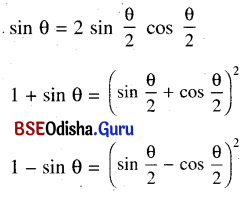(b) 2 sin2 $$\frac{\theta}{2}$$ = 1 – cos θ
2 cos2 $$\frac{\theta}{2}$$ = 1 + cos θ

(c) tan $$\frac{\theta}{2}$$ = $$\frac{\sin \theta}{1+\cos \theta}=\frac{1-\cos \theta}{\sin \theta}$$

(d) sin θ = 3 sin $$\frac{\theta}{2}$$ – 4 sin3 $$\frac{\theta}{2}$$
cos θ = 4 cos3 $$\frac{\theta}{2}$$ – 3 cos $$\frac{\theta}{2}$$

(e) tan θ = $$\frac{3 \tan \frac{\theta}{2}-\tan ^3 \frac{\theta}{2}}{1-3 \tan ^2 \frac{\theta}{2}}$$

Trigonometric Equations:
(a) Equation involving trigonometric equations of unknown angles are called trigonometric function.
(b) Principle solution: The solution ‘x’ of a trigonometric equation is said to be a principle solution if x ∈ (0, 2π)
(c) The solution considered over the entire set R are called the general solution.
(d) General solution of some standard trigonometric equations.

• sin x = 0 ⇒ x = nπ, n ∈ Z
• cos x = 0 ⇒ x = (2n + 1) $$\frac{\pi}{2}$$, n ∈ Z
• tan x = 0 ⇒ x = nπ, n ∈ Z
• sin x = sin α ⇒ x = nπ + (-1)n, n ∈ Z
• cos x = cos α ⇒ x = 2nπ ± α, n ∈ Z
• tan x = tan α ⇒ x = nπ + α, n ∈ Z
• $$\left.\begin{array}{l} \sin ^2 x=\sin ^2 \alpha \\ \cos ^2 x=\cos ^2 \alpha \\ \tan ^2 x=\tan ^2 \alpha \end{array}\right]$$ ⇒ x = nπ ± α
• $$\left.\begin{array}{l} \cos x=\cos \alpha \\ \text { and } \sin x=\sin \alpha \end{array}\right]$$ ⇒ x = nπ ± α, n ∈ Z

Sine Formula:
In any Δ ABC, $$\frac{a}{\sin A}=\frac{b}{\sin B}=\frac{c}{\sin C}$$ or, $$\frac{\sin \mathrm{A}}{a}=\frac{\sin \mathrm{B}}{b}=\frac{\sin \mathrm{C}}{c}$$ = 2R
∴ a = 2R sin A, b = 2R sin B and c = 2R sin C
Also, sin A = $$\frac{a}{2R}$$, sin B = $$\frac{b}{2R}$$ and sin c = $$\frac{c}{2R}$$

Cosine fromulae:
In any Δ ABC,
(i) a2 = b2 + c2 – 2bc cos A
(ii) b2 = c2 + a2 – 2ca cos B
(iii) c2 = a2 + b2 – 2ab cos C
or, → cos A = $$\frac{b^2+c^2-a^2}{2 b c}$$
→ cos B = $$\frac{c^2+a^2-a^2}{2 c a}$$
→ cos C = $$\frac{a^2+b^2-c^2}{2 a b}$$

Projection formulae:
In any Δ ABC,
(i) a = b sin C + c sin B
(ii) b = c cos A + a cos C
(iii) c = a cos B + b cos A

Tangent formulae (Napier’s Analogy);
In any Δ ABC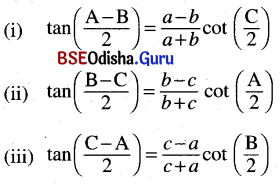Area of Triangle (Heron’s formulae):
(i) Area of triangle ABC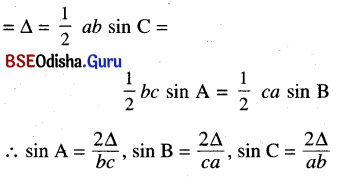(ii) Heron’s formulae:
In any Δ ABC Let 2S = a + b + c
Area of Δ ABC = Δ = $$\sqrt{s(s-a)(s-b)(s-c)}$$
Δ = $$\frac{1}{2}$$ bc sin A = $$\frac{1}{2}$$ ca sin B
= $$\frac{1}{2}$$ ab sin C, Δ = $$\frac{abc}{4R}$$Semi-Angle Formulae: